1. /
2. CBSE
3. /
4. Class 08
5. /
6. Mathematics
7. /
8. NCERT Solutions for Class...

# NCERT Solutions for Class 8 Maths Exercise 8.2

NCERT solutions for Class 8 Maths Comparing Quantities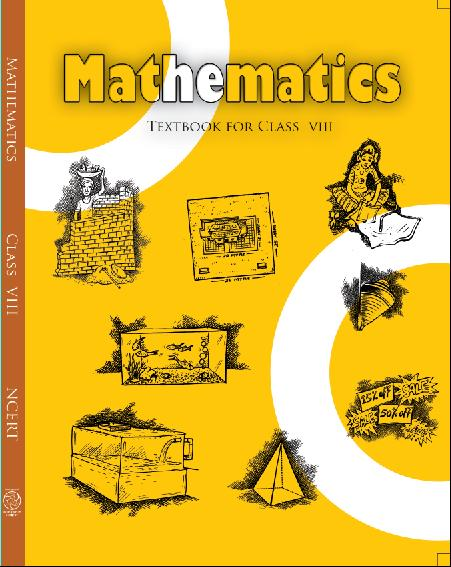## NCERT Solutions for Class 8 Maths Comparing Quantities

###### 1. A man got 10% increase in his salary. If his new salary is Rs.1,54,000, find his original salary.

Ans. Let original salary be Rs.100.

Therefore New salary i.e., 10% increase

= 100 + 10 = Rs.110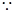New salary is Rs.110, when original salary = Rs.100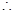New salary is Rs.1, when original salary =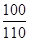New salary is Rs.1,54,000, when original salary =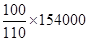= Rs.1,40,000

Hence original salary is Rs. 1,40,000.

NCERT Solutions for Class 8 Maths Exercise 8.2

###### 2. On Sunday 845 people went to the Zoo. On Monday only 169 people went. What is the percent decrease in the people visiting the Zoo on Monday?

Ans. On Sunday, people went to the Zoo

= 845

On Monday, people went to the Zoo = 169

Number of decrease in the people

= 845 – 169 = 676

Decrease percent =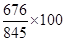= 80%

Hence decrease in the people visiting the Zoo is 80%.

###### 3. A shopkeeper buys 80 articles for Rs.2,400 and sells them for a profit of 16%. Find the selling price of one article.

Ans. No. of articles = 80

Cost Price of articles = Rs. 2,400And Profit

= 16%Cost price of articles is Rs.100, then selling price = 100 + 16 = Rs.116Cost price of articles is Rs.1, then selling price =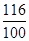Cost price of articles is Rs.2400, then selling price =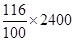= Rs.2784

Hence, Selling Price of 80 articles = Rs.2784

Therefore Selling Price of 1 article

=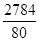= Rs.34.80

###### 4. The cost of an article was Rs.15,500, Rs.450 were spent on its repairs. If it sold for a profit of 15%, find the selling price of the article.

Ans. Here, C.P. = Rs.15,500 and Repair cost = Rs.450

Therefore Total Cost Price = 15500 + 450 = Rs.15,950

Let C.P be Rs.100, then S.P. = 100 + 15

= Rs.115When C.P. is Rs.100, then S.P. = Rs.115When C.P. is Rs.1, then S.P. =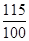When C.P. is Rs.15950, then S.P.

=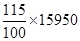= Rs.18,342.50

NCERT Solutions for Class 8 Maths Exercise 8.2

###### 5. A VCR and TV were bought for Rs.8,000 each. The shopkeeper made a loss of 4% on the VCR and a profit of 8% on the TV. Find the gain or loss percent on the whole transaction.

Ans. Cost price of VCR = Rs.8000 and Cost price of TV = Rs.8000

Total Cost Price of both articles

= Rs.8000 + Rs.8000 = Rs. 16,000

Now VCR is sold at 4% loss.

Let C.P. of each article be Rs.100, then S.P. of VCR = 100 – 4 = Rs.96When C.P. is Rs.100, then S.P. = Rs.96When C.P. is Rs.1, then S.P. =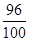When C.P. is Rs.8000, then S.P.

=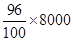= Rs.7,680

And TV is sold at 8% profit, then S.P. of TV = 100 + 8 = Rs.108When C.P. is Rs.100, then S.P. = Rs.108When C.P. is Rs.1, then S.P. =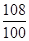When C.P. is Rs.8000, then S.P.

=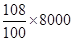= Rs.8,640

Then, Total S.P.

= Rs.7,680 + Rs.8,640 = Rs. 16,320

Since S.P. >C.P.,

Therefore Profit = S.P. – C.P.

= 16320 – 16000 = Rs.320

And Profit% =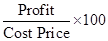=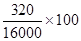= 2%

NCERT Solutions for Class 8 Maths Exercise 8.2

###### 6. During a sale, a shop offered a discount of 10% on the marked prices of all the items. What would a customer have to pay for a pair of jeans marked at Rs.1450and two shirts marked at Rs.850 each?

Ans. Rate of discount on all items = 10%

Marked Price of a pair of jeans = Rs.1450 and Marked Price of a shirt = Rs.850

Discount on a pair of jeans

=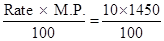= Rs.145S.P. of a pair of jeans = Rs.1450 – Rs.145

= Rs.1305

Marked Price of two shirts =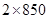= Rs.1700

Discount on two shirts =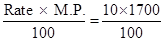= Rs.170S.P. of two shirts = Rs.1700 – Rs.170

= Rs.1530

Therefore the customer had to pay

= 1305 + 1530

= Discount on a pair of jeans

== Rs.145S.P. of a pair of jeans

= Rs.1450 – Rs.145 = Rs.2,835

NCERT Solutions for Class 8 Maths Exercise 8.2

###### 37. A milkman sold two of his buffaloes for Rs.20,000 each. On one he made a gain of 5% and on the other a loss of 10%. Find his overall gain or loss. (Hint: Find CP of each)

Ans. S.P. of each buffalo = Rs.20,000

S.P. of two buffaloes =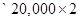= Rs.40,000

One buffalo is sold at 5% gain.

Let C.P. be Rs.100, then S.P. = 100 + 5

= Rs.105When S.P. is Rs.105, then C.P. = Rs.100When S.P. is Rs.1, then C.P. =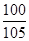When S.P. is Rs.20,000, then C.P.

=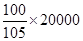= Rs.19,047.62

Another buffalo is sold at 10% loss.

Let C.P. be Rs.100, then S.P. = 100 – 10

= Rs.90When S.P. is Rs.90, then C.P. = Rs.100When S.P. is Rs.1, then C.P. =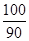When S.P. is Rs.20,000, then C.P.

=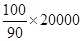= Rs.22,222.22

Total C.P. = Rs.19,047.62 + Rs.22,222.22

= Rs.41,269.84

Since C.P. >S.P.

Therefore here it is loss.

Loss = C.P. – S.P.

= Rs.41,269.84 – Rs. 40,000.00 = Rs.1,269.84

NCERT Solutions for Class 8 Maths Exercise 8.2

###### 8. The price of a TV is Rs.13,000. The sales tax charged on it is at the rate of 12%. Find the amount that Vinod will have to pay if he buys it.

Ans. C.P. = Rs.13,000 and S.T. rate = 12%

Let C.P. be Rs.100, then S.P. for purchaser

= 100 + 12 = Rs.112When C.P. is Rs.100, then S.P. = Rs.112When C.P. is Rs.1, then S.P. =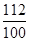When C.P. is Rs.13,000, then S.P.

=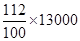= Rs.14,560

NCERT Solutions for Class 8 Maths Exercise 8.2

###### 9. Arun bought a pair of skates at a sale where the discount given was 20%. If the amount he pays is Rs.1,600, find the marked price.

Ans. S.P. = Rs.1,600 and Rate of discount

= 20%

Let M.P. be Rs.100, then S.P. for customer

= 100 – 20 = Rs.80When S.P. is Rs.80, then M.P. = Rs.100When S.P. is Rs.1, then M.P. =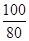When S.P. is Rs.1600, then M.P.

=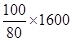= Rs.2,000

NCERT Solutions for Class 8 Maths Exercise 8.2

###### 10. I purchased a hair-dryer for Rs.5,400 including 8% VAT. Find the price before VAT was added.

Ans. C.P. = Rs.5,400 and Rate of VAT = 8%

Let C.P. without VAT is Rs. 100, then price including VAT = 100 + 8 = Rs.108When price including VAT is Rs.108, then original price = Rs.100When price including VAT is Rs.1, then original price =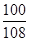When price including VAT is Rs.5400, then original price =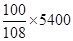= Rs.5000

## NCERT Solutions for Class 8 Maths Exercise 8.2

NCERT Solutions Class 8 Mathematics PDF (Download) Free from myCBSEguide app and myCBSEguide website. Ncert solution class 8 Mathematics includes text book solutions from Class 8 Maths Book . NCERT Solutions for CBSE Class 8 Maths have total 16 chapters. 8 Maths NCERT Solutions in PDF for free Download on our website. Ncert class 8 solutions PDF and Maths ncert class 8 PDF solutions with latest modifications and as per the latest CBSE syllabus are only available in myCBSEguide.

## CBSE app for Class 8

To download NCERT Solutions for class 8 Social Science, Computer Science, Home Science,Hindi ,English, Maths Science do check myCBSEguide app or website. myCBSEguide provides sample papers with solution, test papers for chapter-wise practice, NCERT solutions, NCERT Exemplar solutions, quick revision notes for ready reference, CBSE guess papers and CBSE important question papers. Sample Paper all are made available through the best app for CBSE students and myCBSEguide website.Test Generator

Create question paper PDF and online tests with your own name & logo in minutes.myCBSEguide

Question Bank, Mock Tests, Exam Papers, NCERT Solutions, Sample Papers, Notes

### 25 thoughts on “NCERT Solutions for Class 8 Maths Exercise 8.2”

1. Nice

3. very good ,But some solution is not good.

4. Very Good.
I would like to open this website

5. Nice

6. good

7. Very nice

8. Very grateful

9. Helped alot??

10. Class 8 maths ex8?2 chapter 8 11 sum which is new Edition is not done

11. Thanks so much

12. Very bad.It is not useful

13. Good solutions

14. Nice i understanding it well

15. Good very nice answers can trust ??

16. Excellent website

18. ? nice…..

19. These. Questions are very long plz share short answers

20. Very nice

21. Excellent, fantastic ,marvellous

22. Nice site

23. Gobar solution gu kha Lo salo

24. Gobar jaisa solution gu kha Lo salo

25. Bakchod saale